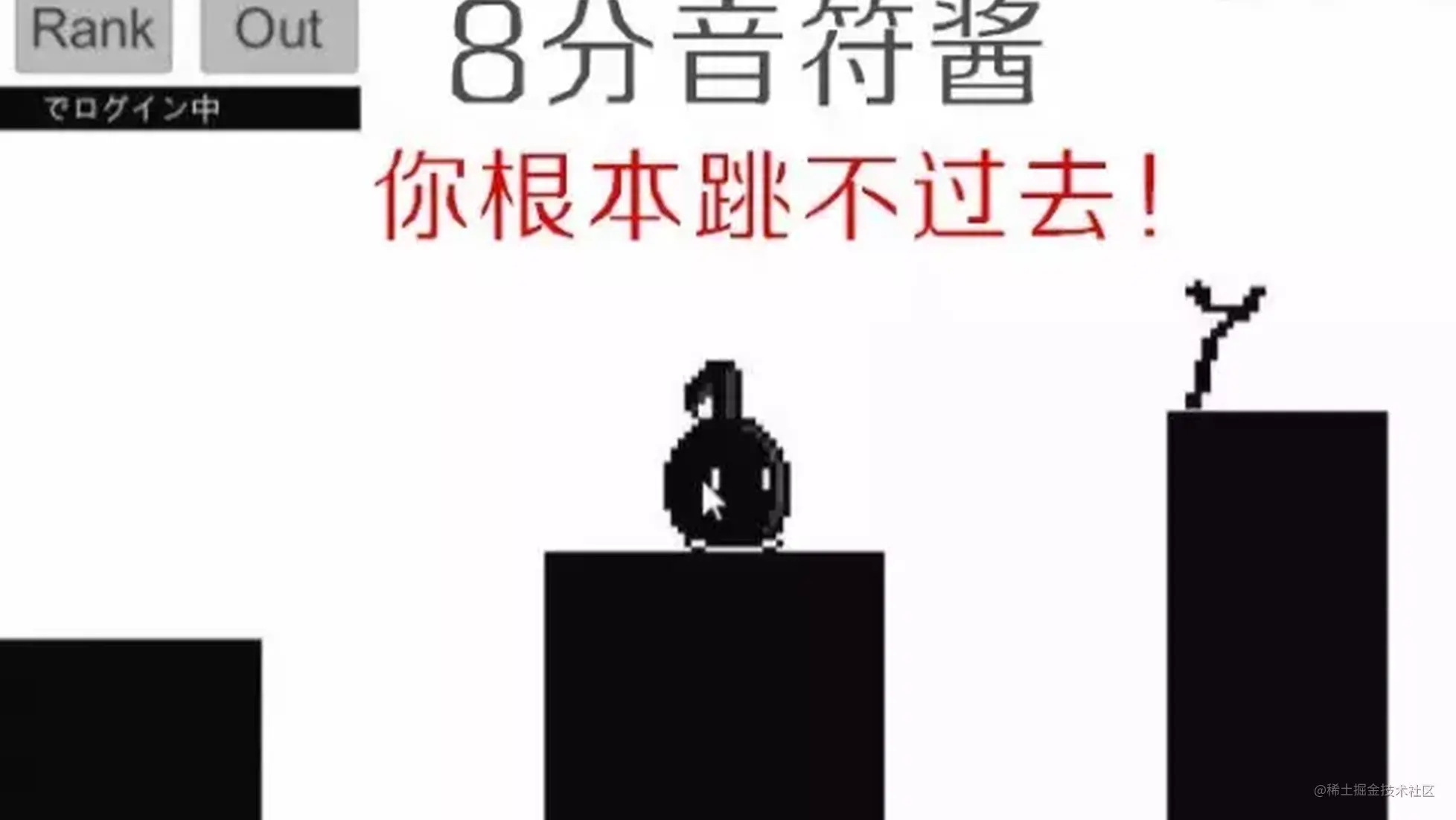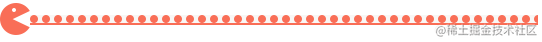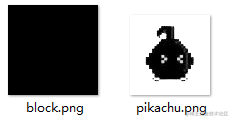# 【Pygame实战】笑到颤抖的小游戏《不要停下来，八音符酱》，边撕边吼边泪奔！

## 导语## 正文1.1 游戏规则

1.2 素材图片1.1 小介绍

Cocos2d模块

``````Cocos2d是一个用于构建2D游戏、演示和其他图形/交互应用程序的框架。

Pyaudio模块：

``````Pyaudio库，使用这个可以进行录音，播放，生成wav文件等等。PyAudio 提供了 PortAudio 的

Python 语言版本，这是一个跨平台的音频 I/O 库，使用 PyAudio 你可以在 Python 程序中播放和录制音频。为

PoTaTudio提供Python绑定，跨平台音频I/O库。使用PyAudio，您可以轻松地使用Python在各种平台上播放和录制

1.2  配置环境

``````pip  install +模块名 或带豆瓣镜像源 pip install -i https://pypi.douban.com/simple/ +模块名

3.1 定义小黑八音符类：

``````import cocos

'''皮卡丘类'''
class Pikachu(cocos.sprite.Sprite):
def __init__(self, imagepath, **kwargs):
super(Pikachu, self).__init__(imagepath)
# 锚点
self.image_anchor = 0, 0
# 初始重置
self.reset(False)
# 更新
self.schedule(self.update)
'''声控跳跃'''
def jump(self, h):
if self.is_able_jump:
self.y += 1
self.speed -= max(min(h, 10), 7)
self.is_able_jump = False
'''着陆后静止'''
def land(self, y):
if self.y > y - 25:
self.is_able_jump = True
self.speed = 0
self.y = y
'''更新(重力下降)'''
def update(self, dt):
self.speed += 10 * dt
self.y -= self.speed
if self.y < -85:
self.reset()
'''重置'''
def reset(self, flag=True):
if flag: self.parent.reset()
# 是否可跳跃
self.is_able_jump = False
# 速度
self.speed = 0
# 位置
self.position = 80, 280

3.2 定义block类：

``````import cocos
import random

'''地面块'''
class Block(cocos.sprite.Sprite):
def __init__(self, imagepath, position, **kwargs):
super(Block, self).__init__(imagepath)
self.image_anchor = 0, 0
x, y = position
if x == 0:
self.scale_x = 4.5
self.scale_y = 1
else:
self.scale_x = 0.5 + random.random() * 1.5
self.scale_y = min(max(y - 50 + random.random() * 100, 50), 300) / 100.0
self.position = x + 50 + random.random() * 100, 03.3 实现游戏主循环：

``````import cfg
import cocos
import struct
from modules import *
from cocos.sprite import Sprite
from pyaudio import PyAudio, paInt16

'''定义声控游戏类'''
class VCGame(cocos.layer.ColorLayer):
def __init__(self):
super(VCGame, self).__init__(255, 255, 255, 255, 800, 600)
# frames_per_buffer
self.num_samples = 1000
# 声控条
self.vbar = Sprite(cfg.BLOCK_IMAGE_PATH)
self.vbar.position = 20, 450
self.vbar.scale_y = 0.1
self.vbar.image_anchor = 0, 0
# 皮卡丘
self.pikachu = Pikachu(cfg.PIKACHU_IMAGE_PATH)
# 地面
self.floor = cocos.cocosnode.CocosNode()
position = 0, 100
for i in range(120):
b = Block(cfg.BLOCK_IMAGE_PATH, position)
position = b.x + b.width, b.height
# 声音输入
audio = PyAudio()
self.stream = audio.open(format=paInt16, channels=1, rate=int(audio.get_device_info_by_index(0)['defaultSampleRate']), input=True, frames_per_buffer=self.num_samples)
# 屏幕更新
self.schedule(self.update)
'''碰撞检测'''
def collide(self):
diffx = self.pikachu.x - self.floor.x
for b in self.floor.get_children():
if (b.x <= diffx + self.pikachu.width * 0.8) and (diffx + self.pikachu.width * 0.2 <= b.x + b.width):
if self.pikachu.y < b.height:
self.pikachu.land(b.height)
break
'''定义游戏规则'''
def update(self, dt):
# 获取每帧的音量
k = max(struct.unpack('1000h', audio_data))
self.vbar.scale_x = k / 10000.0
if k > 3000:
self.floor.x -= min((k / 20.0), 150) * dt
# 皮卡丘跳跃
if k > 8000:
self.pikachu.jump((k - 8000) / 1000.0)
# 碰撞检测
self.collide()
'''重置'''
def reset(self):
self.floor.x = 0

'''run'''
if __name__ == '__main__':
cocos.director.director.init(caption="xiaohei Go Go Go ")
cocos.director.director.run(cocos.scene.Scene(VCGame()))## 总结• 今天的木木子呢，也试玩了一下这个八分音符酱，因为是实在害怕同事会打我，所以就回家录了一下。

• 没能通关，也没办法这个游戏实在不是我能驾驭的，玩了几局嗓子就要开始冒烟了~

• 在这提醒好奇心比较强的宝宝们，一定不要在宿舍，夜晚，玩这个声控小游戏。否则，真的可能会被打！！

### 🎉往期游戏文章推荐：

1.1Pygame实战：爆肝！几千行代码实现《机甲闯关冒险游戏》，太牛了！（❤️建议收藏起来慢慢学❤️）

## 👑文章汇总——

1.1Python—2021 |已有文章汇总 | 持续更新，直接看这篇就够了~